# the values of g ( − 2 ) , g ( 0 ) and g ( 7 )### Precalculus: Mathematics for Calcu...

6th Edition
Stewart + 5 others
Publisher: Cengage Learning
ISBN: 9780840068071### Precalculus: Mathematics for Calcu...

6th Edition
Stewart + 5 others
Publisher: Cengage Learning
ISBN: 9780840068071

#### Solutions

Chapter 2.3, Problem 6E

a.

To determine

## To calculate: the values of g(−2),g(0) and g(7)

Expert Solution

g(2)=4g(0)=7g(7)=4

### Explanation of Solution

Given information: the graph of function g is provided,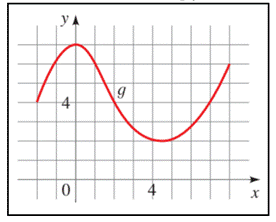Graph: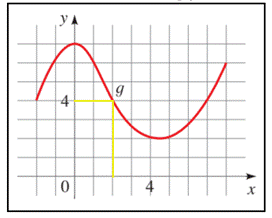Interpretation:

In the graph, the value of g(2) is the corresponding y- value at x = 2 , that is,

g(2)=4

Similarly, the corresponding y -values for,

g(0) at x = 0 is 7

g(7) at x = 7 is 4

Hence,

g(2)=4g(0)=7g(7)=4

b.

To determine

### the domain and the range of the provided graph

Expert Solution

The domain and range of g is [-2,8] and [2,7], respectively.

### Explanation of Solution

Given information: the graph of function g is provided,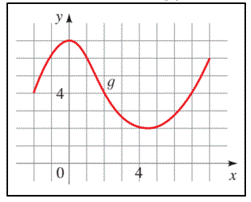Graph:Interpretaion:

The domain of the function g is all the x -values of the points on the graph, and the range is all the corresponding y -values.

From the graph of g , we see that the domain of g is the interval [-2, 8] and the range of g is the interval [2, 7].

Hence, the domain and range of g is [-2,8] and [2,7], respectively.

c.

To determine

### To calculate: the value of x for which g ( x ) = 4

Expert Solution

the value of x for which g ( x ) = 3 is 2,2,7 .

### Explanation of Solution

Given information: the graph of function g is provided,Graph:The function value of g ( a ) from the graph of g , is the height of the graph above the x -axis at x = a ,

Therefore, g ( x ) = 4 is the x -value on the x -axis where the height above it is 4, that are,

g(2)=4g(2)=4g(7)=4

hence, the value of x for which g ( x ) = 3 is 2,2,7 .

d.

To determine

### To calculate: the values for g(x)>4

Expert Solution

the values of x for g(x)>4 are 1x1 and x = 8

### Explanation of Solution

Given information: the graph of function h is provided,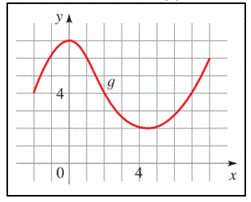Graph: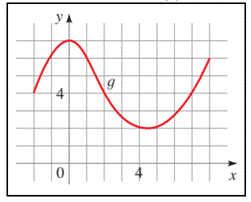Interpretation:

When g(x)>4 is expressed in words it means, g ( x ) is more than to 4. From the graph, write all the x -values whose corresponding y -values are more than 4.

Therefore, the x-values from the graph for g(x)>4 ,

g(1),g(0),g(1),g(8)

Hence, the values of x for g(x)>4 are 1x1 and x = 8

### Have a homework question?

Subscribe to bartleby learn! Ask subject matter experts 30 homework questions each month. Plus, you’ll have access to millions of step-by-step textbook answers!